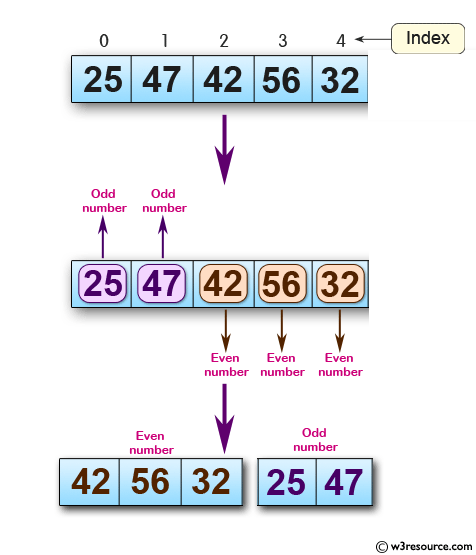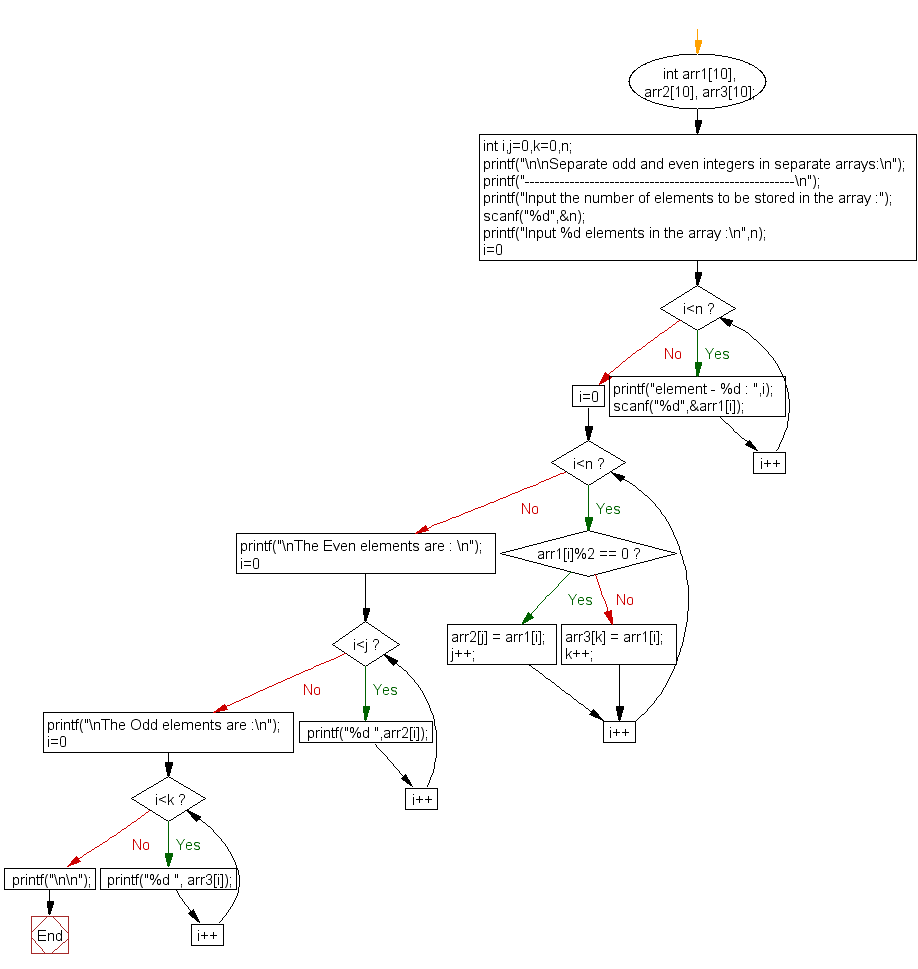﻿ C exercises: Separate odd and even integers in separate arrays - w3resource# C Exercises: Separate odd and even integers in separate arrays

## C Array: Exercise-10 with Solution

Write a program in C to separate odd and even integers in separate arrays.

Pictorial Presentation:Sample Solution:

C Code:

``````#include <stdio.h>

void main()
{
int arr1, arr2, arr3;
int i,j=0,k=0,n;

printf("\n\nSeparate odd and even integers in separate arrays:\n");
printf("------------------------------------------------------\n");

printf("Input the number of elements to be stored in the array :");
scanf("%d",&n);

printf("Input %d elements in the array :\n",n);
for(i=0;i<n;i++)
{
printf("element - %d : ",i);
scanf("%d",&arr1[i]);
}

for(i=0;i<n;i++)
{
if (arr1[i]%2 == 0)
{
arr2[j] = arr1[i];
j++;
}
else
{
arr3[k] = arr1[i];
k++;
}
}

printf("\nThe Even elements are : \n");
for(i=0;i<j;i++)
{
printf("%d ",arr2[i]);
}

printf("\nThe Odd elements are :\n");
for(i=0;i<k;i++)
{
printf("%d ", arr3[i]);
}
printf("\n\n");
}
```
```

Sample Output:

```Separate odd and even integers in separate arrays:
------------------------------------------------------
Input the number of elements to be stored in the array :5
Input 5 elements in the array :
element - 0 : 25
element - 1 : 47
element - 2 : 42
element - 3 : 56
element - 4 : 32

The Even elements are :
42 56 32
The Odd elements are :
25 47
```

Flowchart:C Programming Code Editor:

Improve this sample solution and post your code through Disqus.

What is the difficulty level of this exercise?

﻿